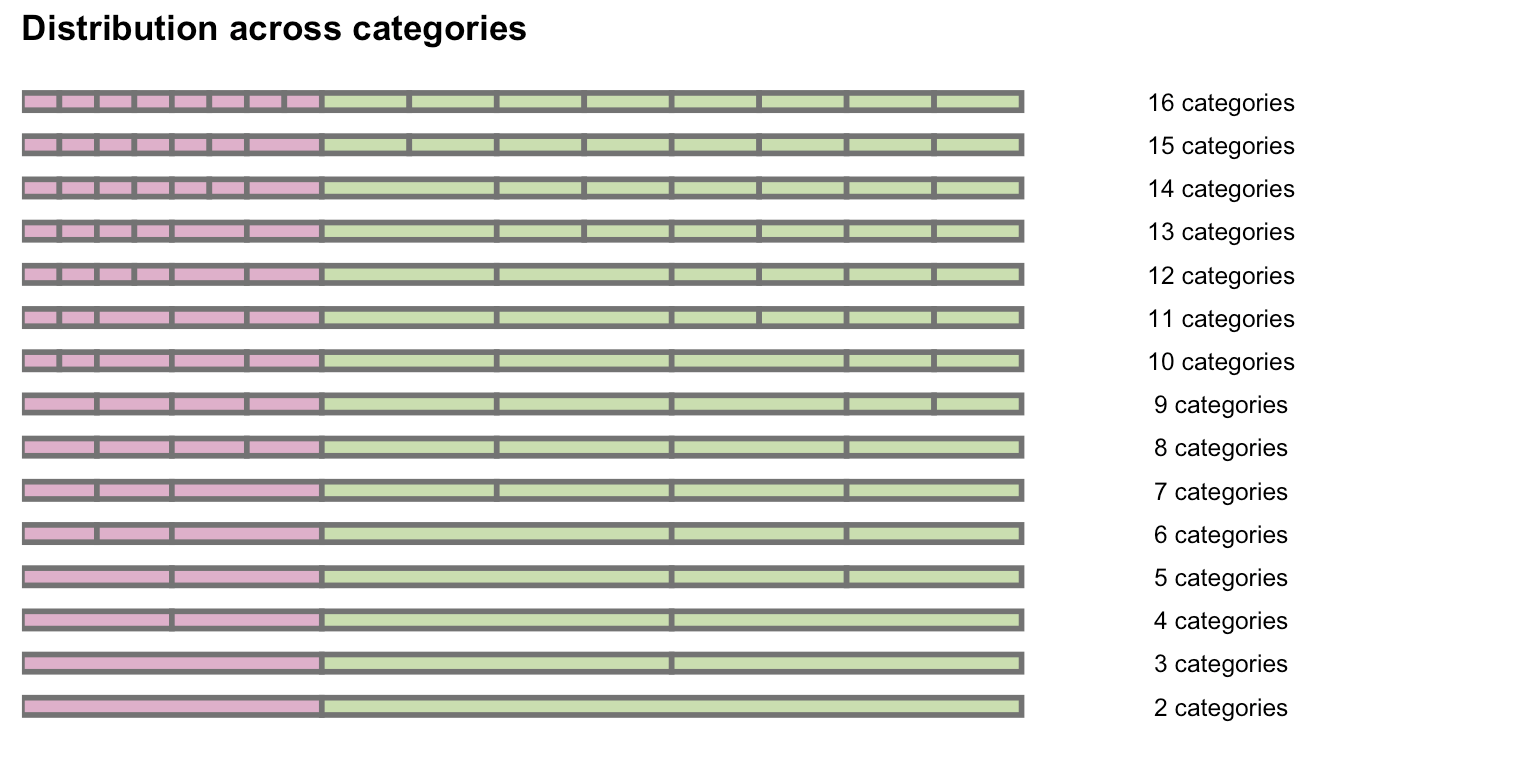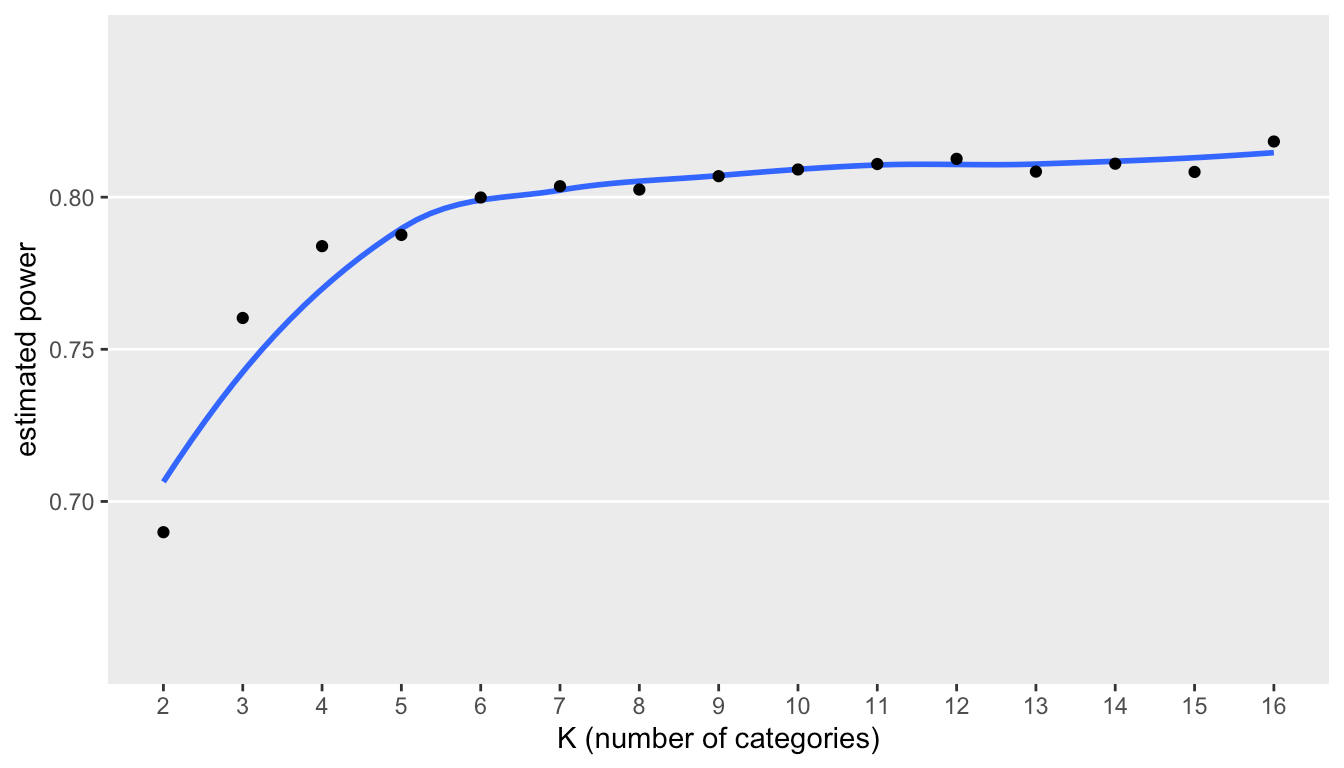Want to share your content on R-bloggers? click here if you have a blog, or here if you don't.

In two Covid-19-related trials I’m involved with, the primary or key secondary outcome is the status of a patient at 14 days based on a World Health Organization ordered rating scale. In this particular ordinal scale, there are 11 categories ranging from 0 (uninfected) to 10 (death). In between, a patient can be infected but well enough to remain at home, hospitalized with milder symptoms, or hospitalized with severe disease. If the patient is hospitalized with severe disease, there are different stages of oxygen support the patient can be receiving, such as high flow oxygen or mechanical ventilation.

It is common to analyze ordinal categorical outcomes like the WHO status measure using a cumulative proportional odds model. (I’ve described these models in a number of posts, starting here and here.) We’ve be been wrestling with the question of whether to use the full 11-point scale or to collapse categories to create a simpler outcome of four or five groups. One issue that comes up is whether this reduction would increase or decrease our ability to detect a treatment effect, assuming of course that there is a treatment effect. To explore the issue, I turned to simulation.

### A very quick recap of the model

In the cumulative proportional odds model, we are comparing a series of cumulative odds across two groups, and we make an assumption that the ratio of these cumulative odds for the two groups is consistent throughout, the proportional odds assumption.

The cumulative odds for the control group that the status is $$x$$ is

$\text{cOdds}_{c}(x) = \frac{P(Status \le x | rx = Control)}{P(Status \gt x |rx = Control)}$

And the cumulative odds ratio comparing Control to Treated is

$\text{COR}_{ct}(x) = \frac{\text{cOdds}_c(x)}{\text{cOdds}_t(x)}$

In the proportional odds model, with a measure that has $$K$$ levels we make the assumption that

$\text{COR}_{ct}(1) = \text{COR}_{ct}(2) = \dots =\text{COR}_{ct}(K)$

The model that we estimate is

$\text{logit}(P(Status \le x)) = \alpha_x – \beta * I(rx = Treat)$ where $$\alpha_x$$ is the log cumulative odds for a particular levels $$x$$, and $$-\beta = \text{COR}_{ct}(k)$$, the (proportional) log odds ratio across all $$k$$ status levels.

### Conceputalizing the categories

I am comparing estimates of models for outcome scales that use a range of categories, from 2 to 16. (I expanded beyond 11 to get a better sense of the results when the gradations become quite fine.) The figure shows the 16-group structure collapsing into 2 groups. The first row depicts the distribution of the control group across 16 categories. The second row combines the 2 rightmost purple categories of the first row into a single category, resulting in 15 total categories. Moving downwards, a pair of adjacent categories are combined at each step, until only 2 categories remain at the bottom.And here are the actual probabilities for the bottom seven rows, from 8 categories down to 2:

baseprobs[7:1]
## []
##  0.075 0.075 0.075 0.075 0.175 0.175 0.175 0.175
##
## []
##  0.075 0.075 0.150 0.175 0.175 0.175 0.175
##
## []
##  0.075 0.075 0.150 0.350 0.175 0.175
##
## []
##  0.150 0.150 0.350 0.175 0.175
##
## []
##  0.15 0.15 0.35 0.35
##
## []
##  0.30 0.35 0.35
##
## []
##  0.3 0.7

### Generating the data

To simulate the data, I use the function genOrdCat in simstudy that uses the base probabilities and the log-odds ratio transforming variable, which in this case is $$z$$. (I introduced this function a while back.) In this case the log odds ratio $$(-\beta)$$ is 1, which translates to a cumulative odds ratio of $$exp(1) = 2.72$$.

library(simstudy)

defA <- defDataAdd(varname = "z", formula = "-1.0 * rx", dist = "nonrandom")

genDT <- function(nobs, baseprobs, defA) {

dT <- genData(nobs)
dT <- trtAssign(dT, grpName = "rx")

dT <- genOrdCat(dT, adjVar = "z", baseprobs, catVar = "r")
dT[]
}

A single data set of 5000 observations with 6 categories looks like this:

set.seed(7891237)
(dx <- genDT(5000, baseprobs[], defA ))
##         id rx  z r
##    1:    1  0  0 1
##    2:    2  1 -1 3
##    3:    3  0  0 5
##    4:    4  0  0 4
##    5:    5  1 -1 1
##   ---
## 4996: 4996  0  0 3
## 4997: 4997  1 -1 4
## 4998: 4998  0  0 3
## 4999: 4999  1 -1 3
## 5000: 5000  1 -1 4

Here are the distributions by treatment arm:

prop.table(dx[, table(rx, r)], margin = 1)
##    r
## rx       1      2      3      4      5      6
##   0 0.0644 0.0772 0.1524 0.3544 0.1772 0.1744
##   1 0.1792 0.1408 0.2204 0.2880 0.1012 0.0704

Here are the cumulative odds and the odds ratio for a response being 2 or less:

(dcodds <- dx[, .(codds = mean(as.numeric(r) <= 2)/mean(as.numeric(r) > 2)), keyby = rx])
##    rx codds
## 1:  0 0.165
## 2:  1 0.471
dcodds[rx == 1, codds] / dcodds[rx==0, codds]
##  2.85

And here are the cumulative odds and COR for a response being 4 or less.

(dcodds <- dx[, .(codds = mean(as.numeric(r) <= 4)/mean(as.numeric(r) > 4)), keyby = rx])
##    rx codds
## 1:  0  1.84
## 2:  1  4.83
dcodds[rx == 1, codds] / dcodds[rx==0, codds]
##  2.62

The CORs are both close to the true COR of 2.72.

### Running the experiment

I was particularly interested in understanding the impact of increasing the number of categories $$K$$ on the probability of observing a treatment effect (i.e. the power). This required generating many (in this case 10,000) data sets under each scenario defined by the number of categories ranging from 2 to 16, and then estimating a cumulative odds model for each data set. I used the clm function in the ordinal package.

Two functions implement this iteration. analyzeDT generates a data set and returns a model fit. iterate repeatedly calls analyzeDT and estimates power for a particular scenario by calculating the proportion of p-values that are less than 0.05:

library(ordinal)

analyzeDT <- function(nobs, baseprobs, defA) {

dT <- genDT(nobs, baseprobs, defA)
clmFit <- clm(r ~ rx, data = dT)
coef(summary(clmFit))

}

iterate <- function(niter, nobs, baseprobs, defA) {
res <- lapply(1:niter, function(x) analyzeDT(nobs, baseprobs, defA))
mean( sapply(res, function(x) x["rx",  "Pr(>|z|)"]) < 0.05)
}

lapply is used here to cycle through each scenario (for enhanced speed mclapply in the parallel package could be used):

set.seed(1295)
power <- lapply(baseprobs, function(x) iterate(niter = 10000,
nobs = 100, x, defA))

### Effect of K on power

A plot of the estimates suggests a strong relationship between the number of categories and power:In this particular case, it seems apparent there are benefits to increasing from 2 to 6 categories. However, there are slight gains to be had by increasing the number of categories much beyond this; indeed, extending to the full 16 categories may not be worth the trouble, as the gains in power are minimal.

These minimal gains need to be weighed against the potential difficulty in acquiring the finely grained categorical outcomes. In cases where the defined categories are completely objective and are naturally collected as part of an operating environment - as in the WHO scale that might be gathered from an electronic health record - there is no real added burden to maximizing the number of categories. However, if the outcome scores are based on patient responses to a survey, the quality of data collection may suffer. Adding additional categories may confuse the patient and make the data collection process more burdensome, resulting in unreliable responses or even worse, missing data. In this case, the potential gains in power may be offset by poor data quality.